We use cookies to enhance your experience on our website. By continuing to use our website, you are agreeing to our use of cookies. You can change your cookie settings at any time. Find out more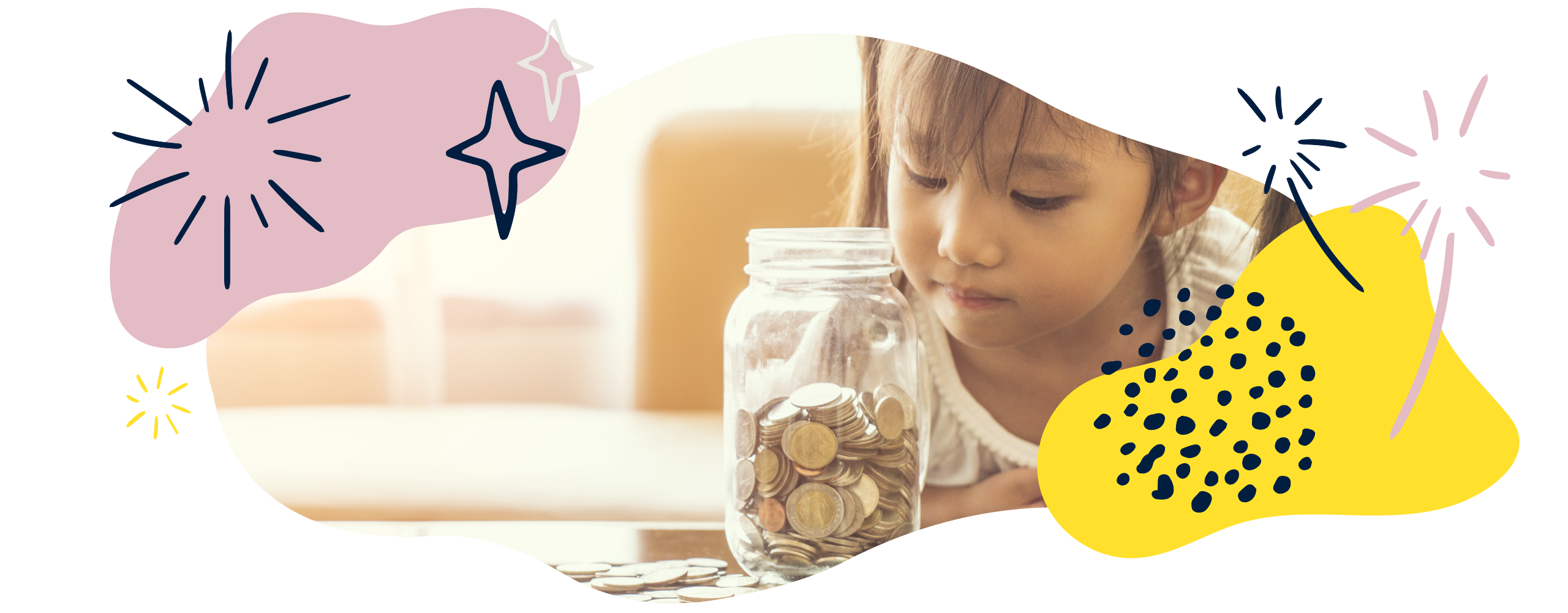# Measurement in Year 4 (Ages 8–9)

In Year 4, children will convert between lots of different standard measures. They will also find the perimeters and areas of shapes, use money, and explore time.

The key words for this section are capacitymass, rectilinear, and volume.

## What your child will learn

Take a look at the National Curriculum expectations for measurement in Year 4 (age 8–9):

#### Convert between different units of measure

Your child will use their understanding of place value, decimals, multiplication, and division to convert units of measure. For example, 3.5km = 3500m because a kilometre is 1000 times bigger than a metre.

They will convert between units of:

• length, such as kilometres to metres
• mass, such as kilograms to grams
• capacity, such as litres to millilitres
• money, such as pounds to pence
• time, such as hours to minutes.

#### Measure and calculate the perimeter of a rectilinear shape

A rectilinear shape is a 2D figure which only has straight lines. All of the edges of a rectilinear shape meet at  right angles. Here are some rectilinear shapes: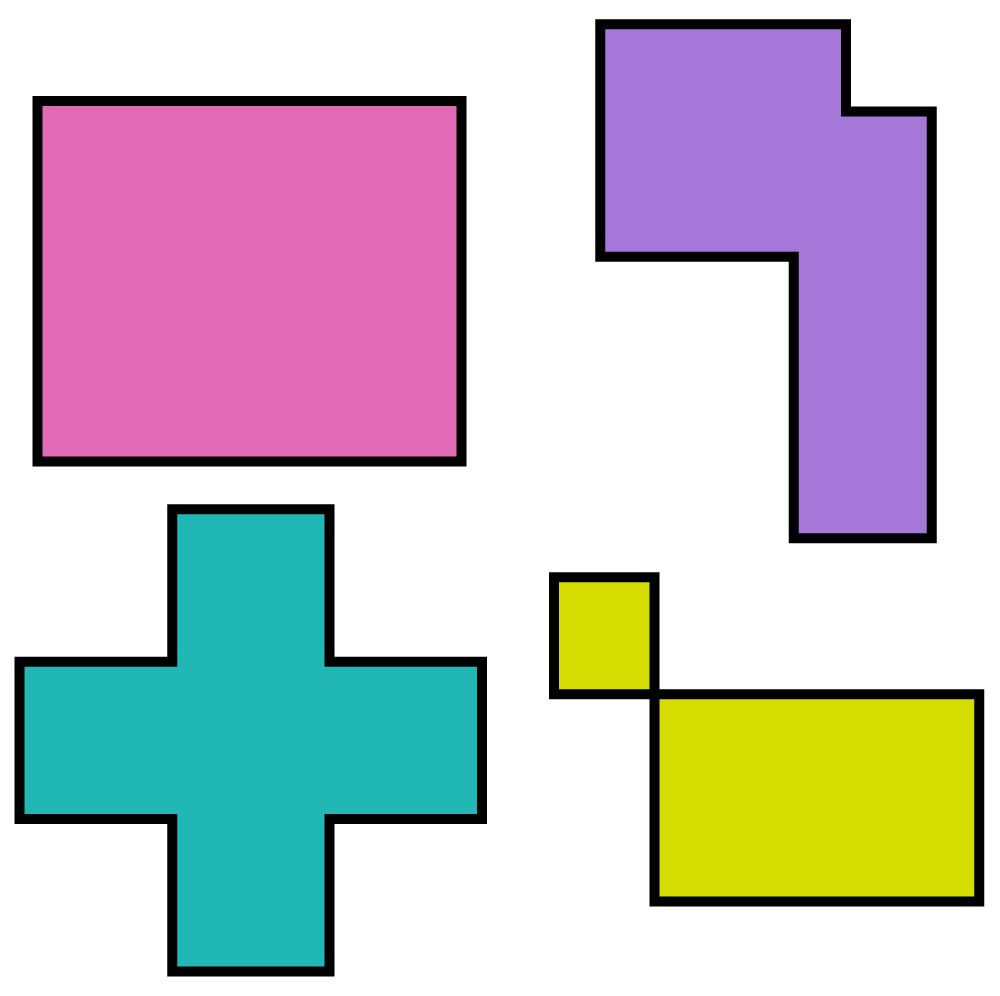Your child will measure and calculate the perimeter of rectilinear shapes. They will record their calculations using units of length such as centimetres and metres.

#### Find the area of rectilinear shapes by counting squares

Your child will find the area of rectilinear shapes. The shapes will be shown on squared paper so, at first, children can count the number of squares to find the area.

Your child will record the area using ‘units squared’. They should be able to relate area to arrays and multiplication. Arrays are objects arranged in equal rows and columns. For example, this is a 6 × 4 array: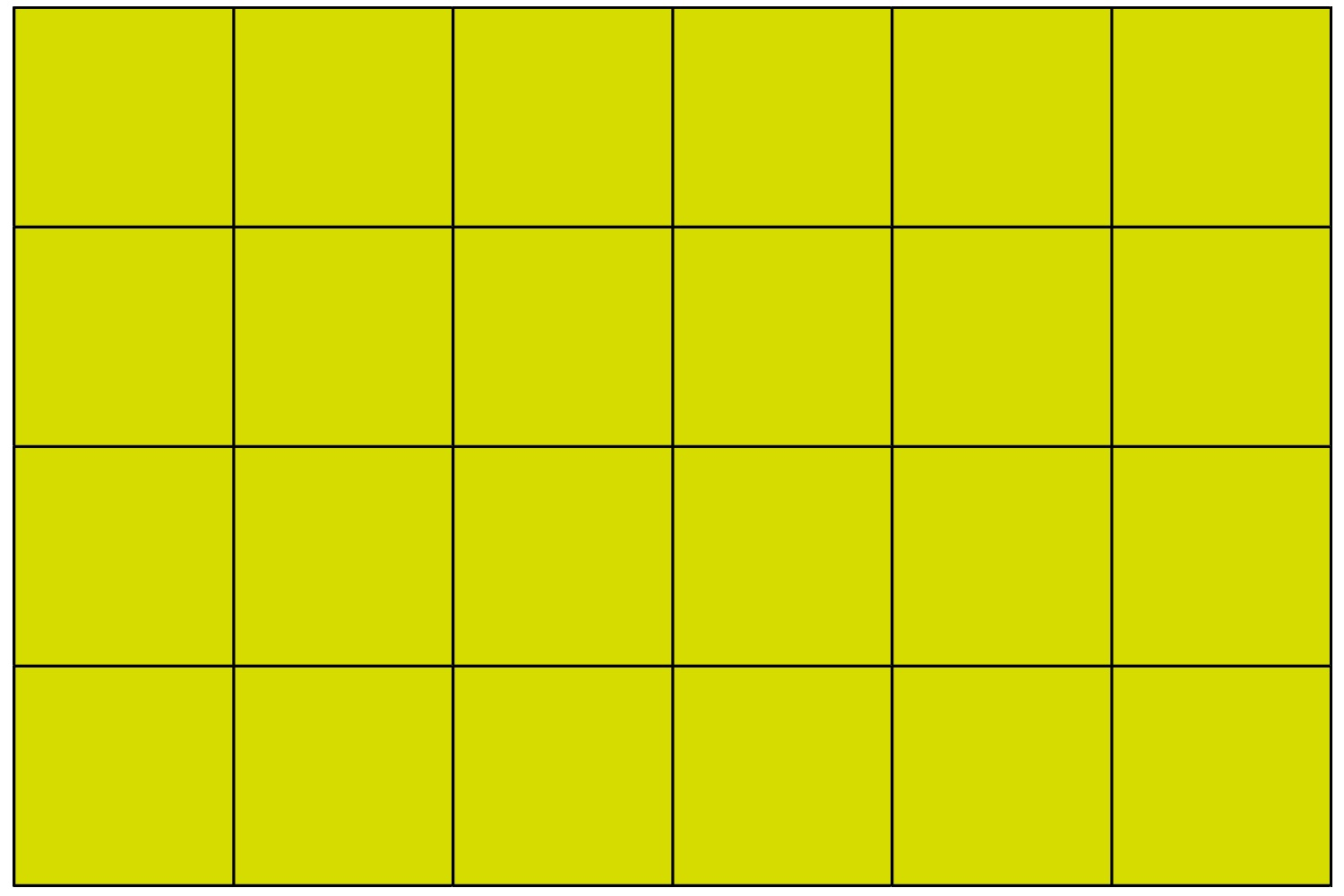The area can be calculated by counting the squares or multiplying the length by the width. In this case, the area would be 6 × 4 = 24 units squared.

#### Calculate with different measures, including money in pounds (£) and pence (p)

Your child will measure, estimate, compare, and calculate with:

• length
• mass
• volume/capacity
• money (in pounds and pence).

This will include multiplying and dividing, as well as recording calculations with the appropriate units.

#### Use and convert time between analogue and digital clocks

Your child will read and write time using analogue clocks, including clocks with Roman numerals from I–XII.

They will also be able to read (and write) the time on digital 12- and 24-hour clocks, and will be able to convert between analogue and digital forms.

#### Convert between units of time

Your child will solve problems involving converting between:

• hours and minutes
• minutes and seconds
• years and months
• weeks and days.

## How to help at home

There are lots of everyday ways you can help your child to understand measurement. Here are just a few ideas.

### 1. Calculate with measures

Try to give your child lots of real-world opportunities to use different units of measurement. For example, you could calculate with money, weigh ingredients, measure objects and distances, record timings, and so on.

To practise measuring distance, throw some bean bags and balls around with your child and measure the distance you can throw and catch the different objects. Your child could record the distances using a table. Can they work out the difference between the distances thrown?

Alternatively, you could find out how fast you can both run 50m. Use a tape measure to measure the distance of 50m and use the stop clock on your phone to find the time taken to run the distance. Compare your times. Discuss the record for the 100m sprint of 9.58 seconds – how far could you run in 9.58 seconds?

Have a go at solving problems, such as:

If one plant measures 1.6 m in height and another measures 132cm, what is the difference in their heights?

To solve this problem, your child will first have to convert the heights into the same units. 1.6m is equivalent to 160cm. Your child can then subtract 132cm from 160cm to find the difference in height of 28cm.

### 2. Explore time on the go

If you get the chance, ask your child to tell the time using analogue clocks, including those with Roman numerals, and digital 12- and 24-hour clocks. Ask your child to read the time on a chosen clock in your home. How would they record this time using an analogue or digital clock? How would they write the time in words?

You could help your child learn to convert units of time by asking questions they can relate to – for instance, if there are three days to go until your birthday, how many hours or minutes are there?

Timetables provide great opportunities for children to solve time-related problems. Find the local bus timetable and ask your child to work out how often the bus will arrive at your local stop. Can they work out the length of a journey using the information in the timetable?

### 3. Explore perimeter

Perimeter means the distance around the outside of a shape. Talk about times in the real world when we need to measure a perimeter, such as putting up a fence in a garden, and look for opportunities to involve your child.

As well as measuring perimeter, your child will calculate missing values in a shape using perimeter. For example:

A rectangle has a perimeter of 34cm.

If the total of the two shorter sides is 12cm, then how long are each of the longer sides?

The total of the two longer sides is 34cm – 12cm = 22cm.

Therefore, each of the longer sides has to be 22cm ÷ 2 = 11cm.

Challenge your child to explore this at home. If the perimeter of your table is 4.50m and each of the shorter sides measures 1m, what must each of the two longer sides measure?

### Activity: Perimeter and area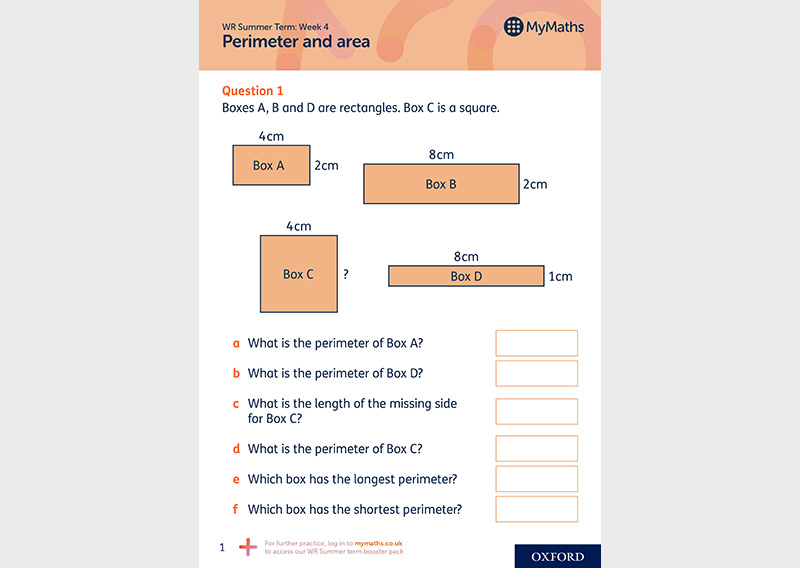Practise calculating area and perimeter.

### 4. Explore area

Area is the space a surface takes up. Help your child look for rectangles around the home – for example, books, tables, and vegetable patches. Can they calculate the area using standard units such as square centimetres (cm²) or square metres (m²)?

Another fun way to get your child thinking about area is to ask them to write their initials using straight lines on 1cm² squared paper, like this: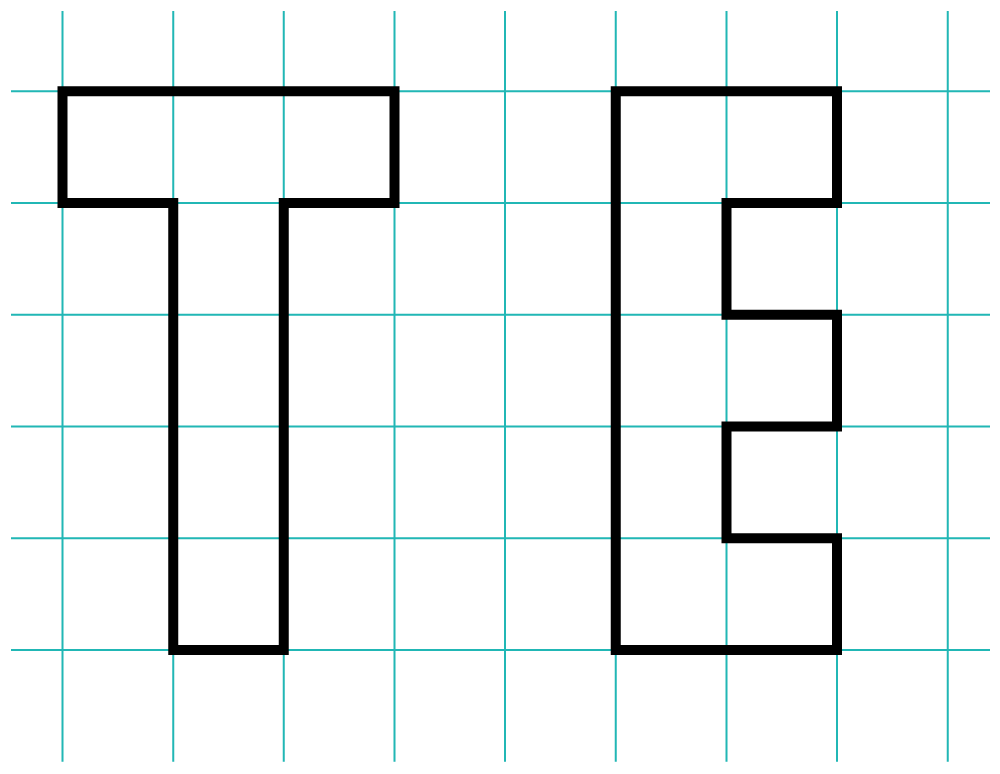They can then estimate which letter has the smallest/largest area (and which has the shortest/longest perimeter). Ask your child to work out the perimeter and area of each letter by counting the squares. Now measure them – were their predictions correct? What can they tell you about each letter?

Note that they should record the perimeter of each letter using cm and the area of each letter using cm².